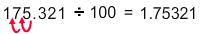# Divide a Decimal Number by Powers of 10

Rate 0 stars Common Core
Quiz size:
Message preview:
Someone you know has shared Divide a Decimal Number by Powers of 10 quiz with you:

To play this quiz, click on the link below:

https://www.turtlediary.com/quiz/divide-a-decimal-number-by-powers-of-10.html

Hope you have a good experience with this site and recommend to your friends too.

Login to rate activities and track progress.
Login to rate activities and track progress.

When dividing by a power of ten, move the decimal point to the left by as many places as there are 0s in the power of 10.

For example, let's calculate:

175.321 ÷ 100

Here, we observe that there are two zeros in 100.

So, move the decimal point two places to the left.So,

175.321 ÷ 100 = 1.75321

ds

A B C D E F G H I J K L M N O P Q R S T U V W X Y Z